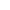Chapter 12 : Area Related to Circles
Q. If the perimeter and the area of a circle are numerically equal, then the radius of the circle is
1. 2 units
2. p units
3. 4 units
4. 7 units
View SolutionQ. The radii of the two circles are 19 cm and 9 cm respectively. Find the radius of the circle which has circumference equal to the sum of the circumferences of the two circles.
View SolutionQ. Fig. depicts an archery target marked with its five scoring regions from the centre outwards as Gold, Red, Blue, Black and White. The diameter of the region representing gold score is 21 cm and each of the other bands is 10.5 cm wide. Find the area of each of the five scoring regionsView SolutionQ. The radii of two circles are 8 cm and 6 cm respectively. Find the radius of the circle having area equal to the sum of the areas of the two circles
View SolutionQ.

Each wheel of a car is of diameter 80 cm. How many complete revolutions does each wheel make in 10 minutes when the car is travelling at a speed of 66 km per hour?

View SolutionQ. Find the area of a sector of a circle with radius 6 cm if angle of the sector is 60.
View SolutionQ.

A horse is tied to a peg at one corner of a square shaped grass field of side 15 m by means of a 5 m long rope. Find
(i) the area of that part of the field in which the horse can graze.
(ii) The increase in the grazing area if the rope were 10 m long instead of 5 m. (Use π=3.14)View SolutionQ. To warn ships for underwater rocks, a lighthouse spreads a red coloured light over a sector of angle 80 to a distance of 16.5 km. Find the area of the sea over which the ships are warned. (Use π=3.14)
View SolutionQ. The length of the minute hand of a clock is 14 cm. Find the area swept by the minute hand in 5 minutes
View SolutionQ. A chord of a circle of radius 10 cm subtends a right angle at the centre. Find the area of the corresponding (i) Minor segment, (ii) Major segment. (use π=3.14)
View SolutionQ. A chord of radius 12 cm subtends an angle of 120 at the centre. Find the area of corresponding segment of the circle. (22/7=3.14 and root 3=1.73).
View SolutionQ. A chord of a circle of radius 15cm subtends an angle of 60 at the centre. Find the areas of the corresponding minor and major segments of the circle.
(Use π=3.14 and 3=1.73)
View SolutionQ. A brooch is made with silver wire in the form of a circle with diameter 35 mm. The wire is also used in making 5 diameters which divide the circle into 10 equal sectors as shown in fig find:
(i) The total length of the silver wire required.
(ii) The arc of each sector of the broochView SolutionQ. In a circle of radius 21 cm, an arc subtends and angle of 60 at the centre. Find:
(i) The length of the arc (ii) Area of the sector formed by the arc (iii) Area of the segment formed by the corresponding chord
View SolutionQ. An umbrella has 8 ribs which are equally spaced . Assuming umbrella to be a flat circle of radius 45 cm, find the area between the two consecutive ribs of the umbrella.View SolutionQ. Find the area of the shaded region in figure, if radii of the two concentric circles with center O are 7 cm and 14 cm respectively and AOC=40.View SolutionQ. Find the area of the shaded region in fig., if PQ=24cm, PR=7cm and O is the centre of the circle.View SolutionQ. Find the area of the shaded region in Fig., if ABCD is a square of side 14cm and APD and BPC are semicircles.View SolutionQ. From each corner of a square of side 4 cm a quadrant of a circle of radius 1 cm is cut and also a circle of diameter 2 cm is cut as shown in fig. Find the area of the remaining portion of the square.View SolutionQ. Find the area of the shaded region in fig, where is circular arc of radius 6 cm has been drawn with vertex O of an equilateral triangle OAB of side 12 cm as centreView Solution